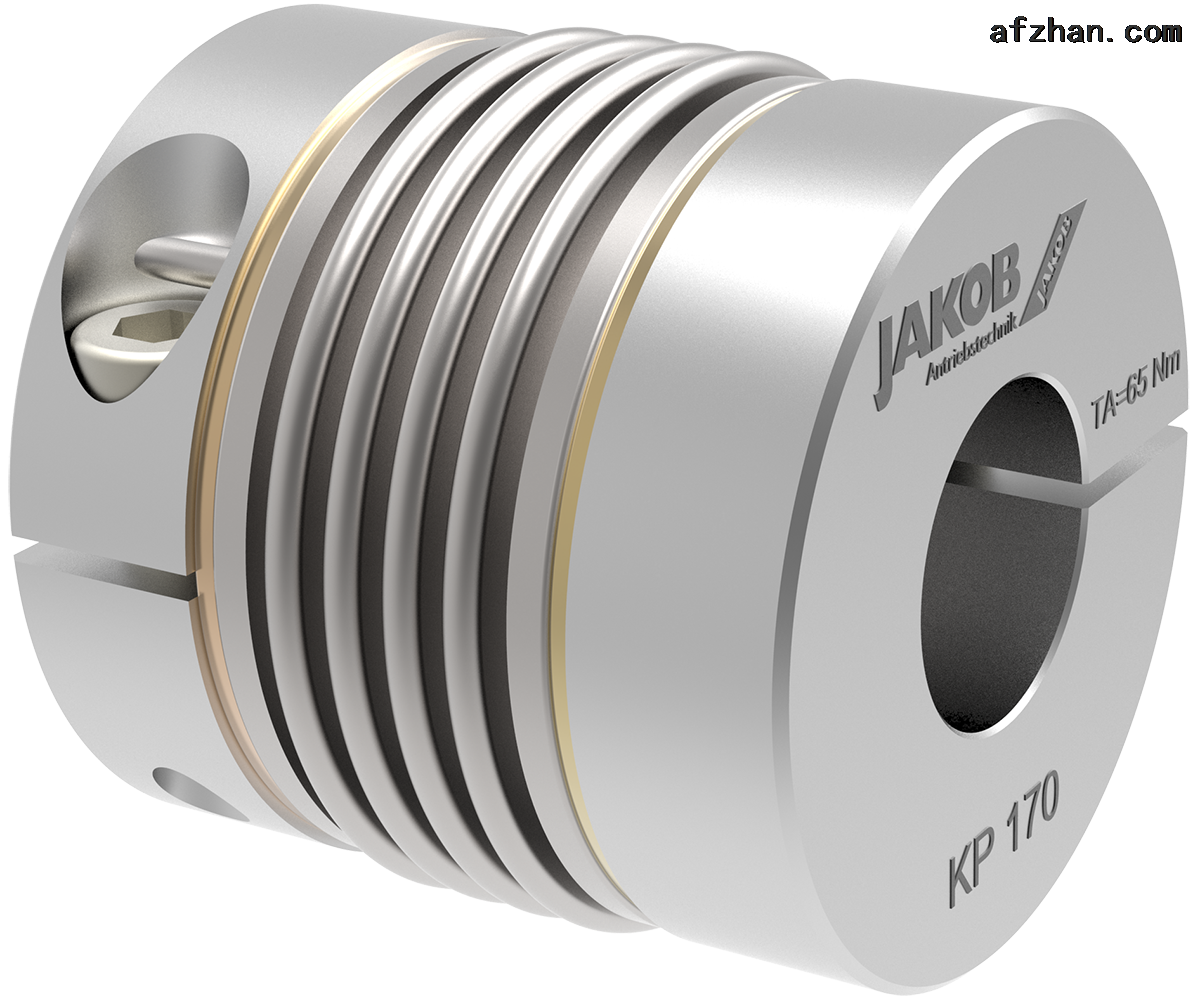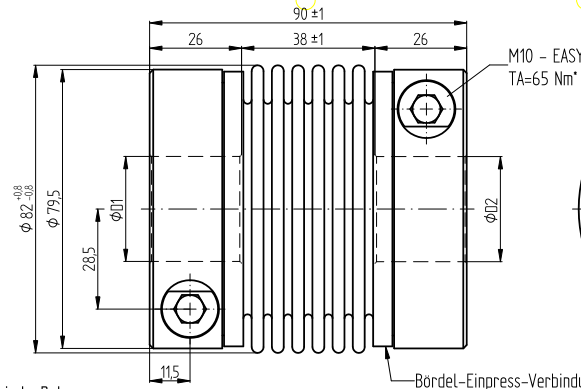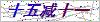# JAKOB KM系列金属波纹管联轴器特点

JAKOB KM系列金属波纹管联轴器特点

6个波纹管--高性价比的标准波纹管系列
-扭矩范围：20-1.300牛米。
-轴的直径：8-85毫米

JAKOB KM系列金属波纹管联轴器特点

6个波纹管--高性价比的标准波纹管系列

-扭矩范围：20-1.300牛米。

-轴的直径：8-85毫米JAKOB金属波纹管联轴器系列KM产品型号及特点。

1JAKOB金属波纹管联轴器KM20

Øa=56c=19f=M6g=30h=8L=70D1=30G6D2=35H6

D1/2最小/最大=Ø8/Ø32mm2JAKOB金属波纹管联轴器KM60

Øa=66c=22f=M8g=33h=9L=77D1=28G6D2=42H6

D1/2最小/最大=Ø13/Ø35mmPoint: MB - 064 07711

3JAKOB金属波纹管联轴器KM80

Øa=82c=28.5f=M10g=38h=11.5L=90D1=30G6D2=42H6

D1/2最小/最大=Ø16/Ø43mmJAKOB金属波纹管联轴器系列KM

6个波纹管--高性价比的标准波纹管系列

-扭矩范围：20-1.300牛米。

-轴的直径：8-85毫米

JAKOB KM系列金属波纹管联轴器特点

JAKOB金属波纹管联轴器KM20Øa=56c=19f=M6g=30h=8L=70D1=30G6D2=35H6

JAKOB金属波纹管联轴器KM60Øa=66c=22f=M8g=33h=9L=77D1=28G6D2=42H6

JAKOB金属波纹管联轴器KM80Øa=82c=28,5f=M10g=38h=11,5L=90D1=30G6D2=42H6

### 留言框

• #### 验证码：请输入计算结果（填写阿拉伯数字），如：三加四=7010-64714988-173

[关闭]